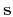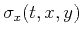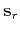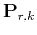Predictive painting of 3-D seismic volumesNext: Predictive painting in 2-D Up: Fomel: Predictive painting Previous: Introduction

# Destruction and prediction of plane waves

Plane-wave destruction originates from a local plane-wave model for characterizing seismic data (Fomel, 2002). The mathematical basis is the local plane differential equation(1)

whereis the wave field andis the local slope, which may also depend onand(Claerbout, 1992). In the case of a constant slope, equation 1 has the simple general solution(2)

whereis an arbitrary waveform. Equation 2 is nothing more than a mathematical description of a plane wave. Assuming that the slopevaries in time and space, one can design a local operator to propagate each trace to its neighbors.

Letrepresent a seismic section as a collection of traces:, wherecorresponds toforA plane-wave destruction operator (Fomel, 2002) effectively predicts each trace from its neighbor and subtracts the prediction from the original trace. In the linear operator notation, the plane-wave destruction operation can be defined as(3)

whereis the destruction residual, andis the destruction operator defined as(4)

wherestands for the identity operator, anddescribes prediction of tracefrom trace. Prediction of a trace consists of shifting the original trace along dominant event slopes. The prediction operator is a numerical solution of equation 1 for local plane wave propagation in thedirection. The dominant slopes are estimated by minimizing the prediction residualusing regularized least-squares optimization. I employ shaping regularization (Fomel, 2007a) for controlling the smoothness of the estimated slope fields. In the 3-D case, a pair of inline and crossline slopes,and, and a pair of destruction operators,and, are required to characterize the 3-D structure. Each prediction in 3-D occurs in either inline or crossline direction and thus conforms to equation 4. However, as explained below in the discussion of Dijkstra's algorithm, it is possible to arrange all 3-D traces in a sequence for further processing.

Prediction of a trace from a distant neighbor can be accomplished by simple recursion. Predicting tracefrom traceis simply(5)

Ifis a reference trace, then the prediction of traceis. I call the recursive operatorpredictive painting. Once the elementary prediction operators in equation 4 are determined by plane-wave destruction, predictive painting can spread information from a given trace to its neighbors recursively by following the local structure of seismic events. The next section illustrates the painting concept using 2-D examples.Predictive painting of 3-D seismic volumesNext: Predictive painting in 2-D Up: Fomel: Predictive painting Previous: Introduction

2013-03-02